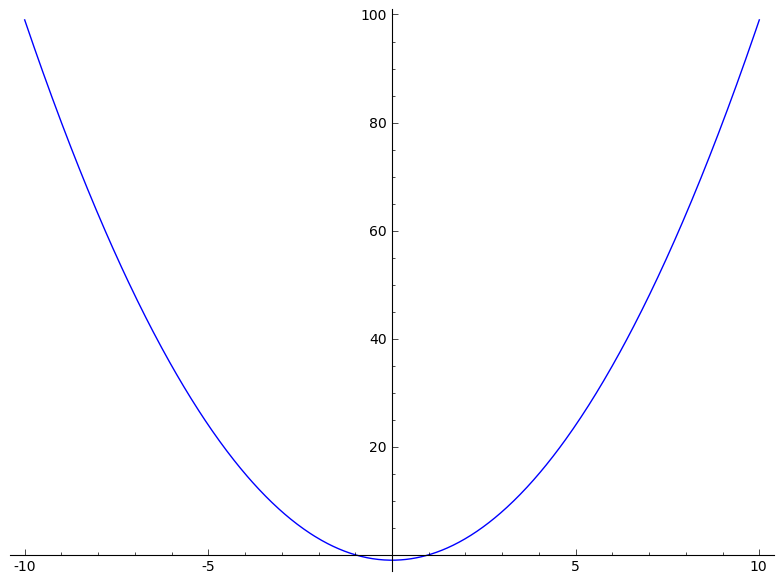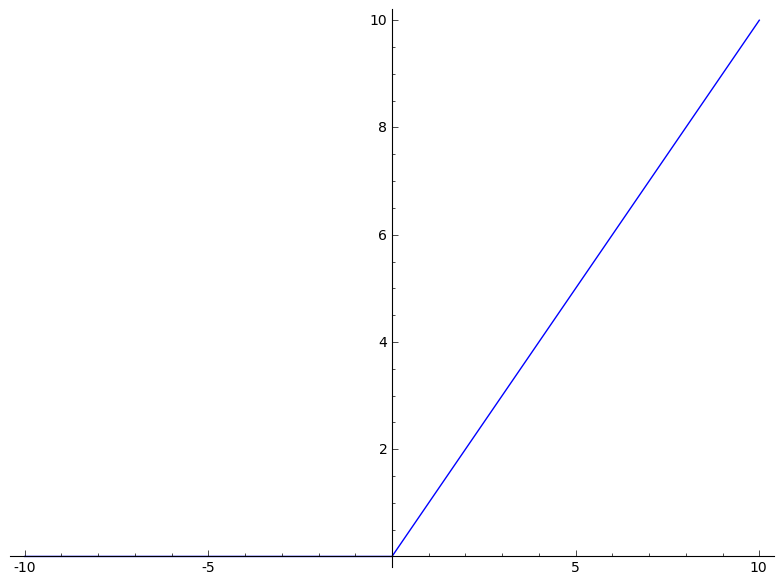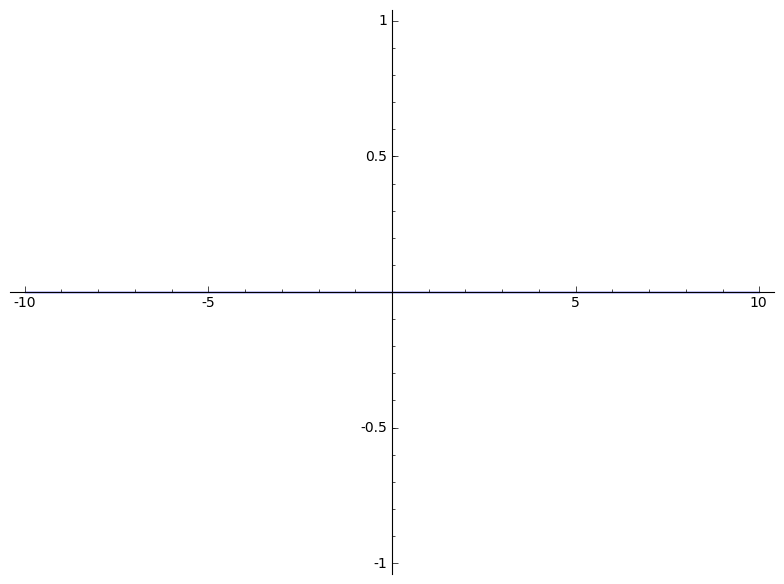Python function vs Symbolic function in Sage

## Python function vs Symbolic function in Sage

21 janvier 2013 | Catégories: sage | View Comments

This message is about differences between a Python function and a symbolic function. This is also explained in the Some Common Issues with Functions page in the Sage Tutorial.

In Sage, one may define a symbolic function like:

sage: f(x) = x^2-1


And draw it using one the following way (both works):

sage: plot(f, (x,-10,10))
sage: plot(f(x), (x,-10,10))Here both f and f(x) are symbolic expressions:

sage: type(f)
<type 'sage.symbolic.expression.Expression'>
sage: type(f(x))
<type 'sage.symbolic.expression.Expression'>


although there are different:

sage: f
x |--> x^2 - 1
sage: f(x)
x^2 - 1


Now if f is a Python function defined with a def statement:

sage: def f(x):
....:     if x>0:
....:         return x
....:     else:
....:         return 0


It is really a Python function:

sage: f
<function f at 0xb933470>
sage: type(f)
<type 'function'>


As above, one can draw the function f:

sage: plot(f, (x,-10,10))But be carefull, drawing f(x) will not work as expected:

sage: plot(f(x), (x,-10,10))Why? Because, the python function f gets evaluated on the variable x and this may either raise an exception depending on the definition of f or return some result which might not be a symbolic expression. Here f(x) gets always evaluated to zero because in the definition of f, bool(x > 0) returns False:

sage: x
x
sage: bool(x > 0)
False
sage: f(x)
0


Hence the following constant function is drawn:

sage: plot(0, (x,-10,10))


which is not what we want.